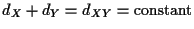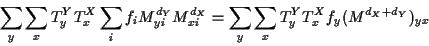Next: Probabilistic Dynamic Programming and Up: Probability of an evolutionary Previous: How to compute this

#### Independence of the position of the root.

It can be shown that the probability of an EC is independent of where we place the root of the tree. This is a direct consequence of the symmetry of the evolution model mentioned in 1.1. To prove this, we first show that the probabilities are not affected if we move the root along the edge liking its two descendants (X and Y). The length of the branch from X to Y will remain constant, we will just change dX and dY so that. The probability of the EC isMoving the summations around and using the symmetry relation of f and M and noticing that the summation in i gives a matrix product, we obtainand the formula is independent of dX and dY, only depends on their sum. By continuity, this can be extended to any position within the EPT. Consequently for the purposes of evaluating the probability of a particular EC, we can place the root wherever it is most convenient.Next: Probabilistic Dynamic Programming and Up: Probability of an evolutionary Previous: How to compute this
Gaston Gonnet
1998-07-14